# Polarization in groups of Bayesian agents

## Abstract

In this paper we present the results of a simulation study of credence developments in groups of communicating Bayesian agents, as they update their beliefs about a given proposition p. Based on the empirical literature, one would assume that these groups of rational agents would converge on a view over time, or at least that they would not polarize. This paper presents and discusses surprising evidence that this is not true. Our simulation study shows that these groups of Bayesian agents show group polarization behavior under a broad range of circumstances. This is, we think, an unexpected result, that raises deeper questions about whether the kind of polarization in question is irrational. If one accepts Bayesian agency as the hallmark of epistemic rationality, then one should infer that the polarization we find is also rational. On the other hand, if we are inclined to think that there is something epistemically irrational about group polarization, then something must be off in the model employed in our simulation study. We discuss several possible interfering factors, including how epistemic trust is defined in the model. Ultimately, we propose that the notion of Bayesian agency is missing something in general, namely the ability to respond to higher-order evidence.

This is a preview of subscription content, access via your institution.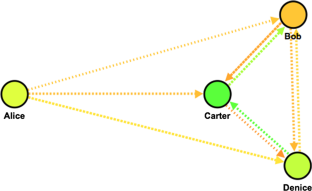Fig. 1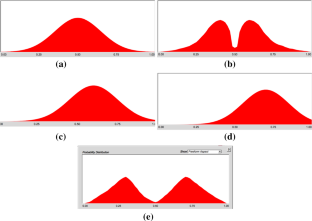Fig. 2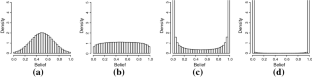Fig. 3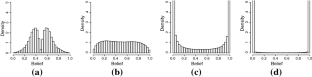Fig. 4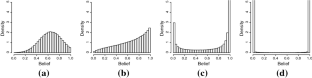Fig. 5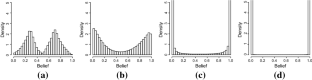Fig. 6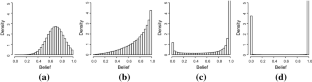Fig. 7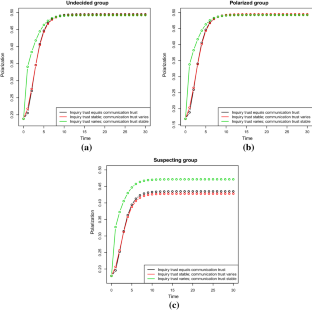Fig. 8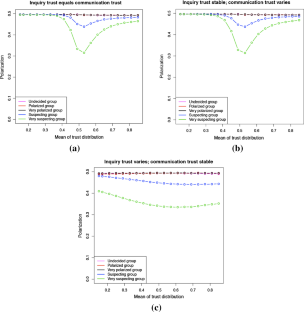Fig. 9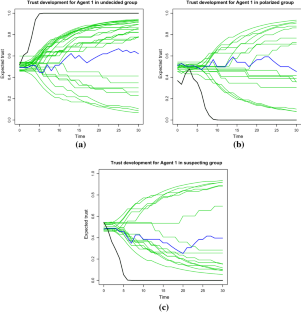Fig. 10Fig. 11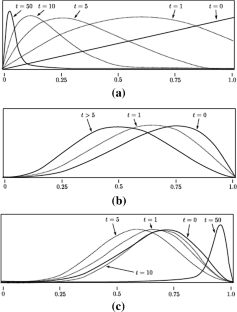Fig. 12
1. 1.

We understand Bayesian agents as agents that update their degrees of belief using conditional probabilities. We will expand on the specifics of the agents that we simulated in Sect. 3.

2. 2.

In general, we are talking groups of agents that are investigating or exchanging information about some proposition p.

3. 3.

Other possible accounts of epistemic rationality could be dynamic epistemic logic or epistemic utility theory, but we will focus on the Bayesian approach here.

4. 4.

These are subjective probabilities, i.e. their degrees of belief in a proposition p are an expression for how likely they think it is that p is true.

5. 5.

In the model, p is assumed to be true. This is an assumption of mathematical convenience in the underlying mathematical model for Laputa. It makes it easier to discuss the epistemic competence of the network. This assumption will not make a difference to our experiment, however.

6. 6.

See Sect. 4.5 for a discussion of the difference between complex deliberation and how communication is understood in the model.

7. 7.

Since we are only considering the proposition p, we have that $$C_\alpha ^{\,t}(p)=1-C_\alpha ^{\,t}(\lnot p)$$.

8. 8.

Conversely, $$1-\tau _{\sigma \alpha }^{\,t}$$ is about $$\alpha$$’s credence in propositions about $$\sigma$$ not being reliable.

9. 9.

This expected value is found by integrating the trust function over all its possible values, but we won’t go into the mathematical details here. See “Appendix A” for more details.

10. 10.

The details of how to get this equation can be found in “Appendix A”.

11. 11.

The results in Sect. 4 pertain to these groups of 50 members, but preliminary testing indicated similar results for groups of 5–25 members.

12. 12.

The competence of the social network, and a lot of the other specifications for setting up a social network in Laputa is specified by probability distributions for the different settings for the group. So the competence of the group is actually determined by a normal distribution with mean 0.6 and standard deviation 0.15. See “Appendix B” for the precise details for how we set up the social networks for our experiment.

13. 13.

The standard deviation is defined as the square root of the variance of a data set or a distribution of data points. The variance is the expected squared deviation of the data points from the average value. Let X be a collection of n data points. When each value is equally likely, the variance, Var(X), is given as $$\frac{1}{n}\sum _{i=1}^n(x_i-\mu )^2$$ where $$\mu$$ is the average value of the data points. The standard deviation is then the square root of this value.

14. 14.

Meaning that the distribution is drawn by hand, making it only approximately symmetrical. We could also have gone for a distribution where there were several subgroups on both sides of the middle, but this is less interesting, since the simulation program only distinguishes between two different, mutually exclusive opinions; either p or not-p.

15. 15.

Because of how the underlying maths of Laputa works, we can expect that a group leaning toward not-p would behave symmetrically to the suspecting and very suspecting groups.

16. 16.

From this point, ‘distributions of trust’ will refer to these distributions of distributions. For simplicity, one can think of them as distributions of expected trust in sources.

17. 17.

It is an upshot of the underlying Bayesian model that once agents reach degree of belief 1 or 0, they can no longer change their minds. We return to this point in Sect. 4.5.

18. 18.

We return to the reason for this stability after approximately 10 time steps in Sect. 4.5.

19. 19.

The pink line isn’t visible in this spot because it has the same value as all of the other graphs in Fig. 9b.

20. 20.

Similar to the tests for trust development in Fig. 10, the data for these tests are collected manually in the simulation environment, meaning that the program does not automatically give statistics about simulations where trust updating is turned off. This means that the results in Figs. 10 and 11 are not statistically significant in the same way as our original experiment in Figs. 3, 4, 5, 6 and 7.

21. 21.

In the model, you can specify a threshold T, which is a degree of certainty in p (or not-p) that $$\beta$$ has to have, before he can communicate that p (or not-p) to $$\alpha$$. See “Appendix A” for more details.

22. 22.

Note, however, that a simulation approach to exchanging arguments within a Bayesian Network structure [put forth by Jern et al. (2014)] showed similar results to our study. The authors conclude that polarization might not be an irrational behavior after all, since it also happens within a Bayesian updating scheme. We discuss this in more detail in Sect. 5.2.

23. 23.

This might not be totally unreasonable, since a person that is completely convinced of something requires extremely compelling evidence to lower her belief, and it is not guaranteed that such evidence even exists. For instance, if I am convinced of the proposition “people cannot fly without assistance”, then I require very compelling evidence to change my mind and that evidence might never come up. But one might have the intuition that there should be a non-zero chance that such evidence exists, which is impossible in the Bayesian framework. In the model, the chance of finding evidence that will change my mind is exactly zero.

24. 24.

See “Appendix A” for the mathematical explanation.

25. 25.

There is an alternative interpretation of the model where a given piece of communication or inquiry represents a novel argument for p (or not-p), instead of just a statement that p (or not-p). See Olsson (2013) for an implementation and discussion of this interpretation of the model. On this interpretation it seems reasonable that agents keep updating their beliefs based on the repeated information from a given source, since the source is giving a new argument for p (or not-p) each time.

26. 26.

Note that a given agent receiving information in the simulation treats all her sources as statistically independent of one another. This is because of an assumption in the underlying mathematical model called source independence, where the agent treats the evidence from her various sources as unconnected. The assumption is there for mathematical convenience, but it is motivated (by the creator of the model) by the fact that we do not usually have conspiracy theories that the different people we talk to are in cahoots to convince us that p (or not-p) is the case (Angere and Olsson 2017, p. 8). We will not discuss the reasonableness of this assumption here, or its applicability to real-life agents, but just note that it has an effect when we start discussing polarization as a case of disagreement between agents. When several, independent sources disagree with a given agent, it is a strong reason to think that the agent, and not the others, is wrong about p. We discuss this possibility in Sect. 5.2.

27. 27.

Remember that in our definition of group polarization, belief polarization is a special case with a group of just two members, cf. Sect. 2.

28. 28.

E.g. biased assimilation (Lord et al. 1979) or (dis)confirmation bias (Taber and Lodge 2006).

29. 29.

Kelly uses the term ‘reasonable’ instead of rational here (Kelly 2008), but his discussion is still relevant for our purposes.

30. 30.

Here, narrow evidence means the same data/or evidence is available to the participants, whereas broad evidence means all of the factors for generating and justifying beliefs, including background assumptions and awareness of alternative explanations of the data (Kelly 2008).

31. 31.

The evidence does get different weight depending on whether the source is trusted or not, but this is not the same as scrutinizing the evidence. The initial distribution in the simulation of which sources are and are not trusted is independent of whether the source says that p or that not-p.

32. 32.

This is the case even if we said that narrow evidence is just the communication that the agents share with each other.

33. 33.

Example: an agent that has above 0.5 credence in p hears from a trusted source (meaning degree of trust is above 0.5) that not-p. Based on the updating formula the agent then lowers both the credence in p and the trust in the source. See “Appendix A” for details.

34. 34.

E.g. the agents are exchanging novel arguments about p and are updating their beliefs based on the number of persuasive arguments available to them (Olsson 2013).

35. 35.

Indeed, p is assumed to be true in the model.

36. 36.

Remember that sources are treated as independent in the model.

37. 37.

Thank you to an unknown reviewer for this suggestion.

38. 38.

If two propositions A and B are independent then the probability of them happening together equals the product of the probabilities of each proposition: $$P(A \wedge B)=P(A)P(B)$$.

39. 39.

See Angere (2010, Appendix A) for the derivation.

40. 40.

When two events A and B are be probabilistically independent means that the probability that they happen together is given as the product of their individual probabilities: $$P(A\wedge B)=P(A)P(B)$$.

41. 41.

See Angere (2010, Appendix A) for the full derivation.

42. 42.

See Angere (2010, Appendix B) for the derivation of the updated trust functions.

43. 43.

The program is developed by Staffan Angere and Eric Olsson at Lund University, Sweden. The newest version of the program is freely available for academic purposes at http://www.luiq.lu.se/software-for-social-epistemology-laputa-1-6-now-available/.

## References

1. Abrams, D., Wetherell, M., Cochrane, S., Hogg, M. A., & Turner, J. C. (1990). Knowing what to think by knowing who you are: Self-categorization and the nature of norm formation, conformity and group polarization. British Journal of Social Psychology, 29(2), 97–119.

2. Anderson, L. R., & Holt, C. A. (1997). Information cascades in the laboratory. The American Economic Review, 87(5), 847–862.

3. Angere, S. (2010). Knowledge in a social network. (unpublished manuscript).

4. Angere, S., & Olsson, E. (2017). Publish late, publish rarely! Network density and group competence in scientific communication. In T. Boyer, C. Mayo-Wilson, & M. Weisberg (Eds.), Scientific collaboration and collective knowledge. Oxford: Oxford University Press.

5. Angere, S., Masterton, G., & Erik, O. (forthcoming). The epistemology of social networks. Oxford: Oxford University Press.

6. Batson, C. D. (1975). Rational processing or rationalization? The effect of disconfirming information on a stated religious belief. Journal of Personality and Social Psychology, 32(1), 176–184.

7. Christensen, D. (2010). Higher-order evidence. Philosophy and Phenomenological Research, 81(1), 185–215.

8. Cook, J., & Lewandowsky, S. (2016). Rational irrationality: Modeling climate change belief polarization using Bayesian networks. Topics in Cognitive Science, 8(1), 160–179.

9. Easwaran, K., Fenton-glynn, L., Hitchcock, C., & Velasco, J. D. (2016). Updating on the credences of others: Disagreement, agreement and synergy. Philosophers’ Imprint, 16, 1–39.

10. Hussain, F. K., Chang, E., & Hussain, O. K. (2007). State of the art review of the existing Bayesian-network based approaches to trust and reputation computation. In Second international conference on internet monitoring and protection, ICIMP 2007. ICIMP.

11. Isenberg, D. J. (1986). Group polarization. A critical review and meta-analysis. Journal of Personality and Social Psychology, 50(6), 1141–1151.

12. Jern, A., Chang, K. M. K., & Kemp, C. (2014). Belief polarization is not always irrational. Psychological Review, 121(2), 206–224.

13. Kelly, T. (2008). Disagreement, dogmatism, and belief polarization. Journal of Philosophy, 105(10), 611–633.

14. Khosravifar, B., Bentahar, J., Gomrokchi, M., & Alam, R. (2012). CRM: An efficient trust and reputation model for agent computing. Knowledge-Based Systems, 30, 1–16.

15. Kolmogorov, A. N. (1933). Grundbegriffe der Wahrscheinlichkeitsrechnung. Berlin: Springer.

16. Kunda, Z. (1990). The case for motivated reasoning. Psychological Bulletin, 108(3), 480–498.

17. Lord, C. G., Ross, L., & Lepper, M. R. (1979). Biased assimilation and attitude polarization: The effects of prior theories on subsequently considered evidence. Journal of Personality and Social Psychology, 37(11), 2098–2109.

18. Ma, H., Turner, J. C., & Davidson, B. (1990). Polarized norms and social frames of reference: A test of the self-categorization theory of group polarization. Basic and Applied Social Psychology, 11(1), 77–100.

19. Mackie, D. M. (1986). Social identification effects in group polarization. Journal of Personality and Social Psychology, 50(4), 720.

20. Miller, A. G., McHoskey, J. W., Bane, C. M., & Dowd, T. G. (1993). The attitude polarization phenomenon: Role of response measure, attitude extremity, and behavioral consequences of reported attitude change. Journal of Personality and Social Psychology, 64(4), 561–574.

21. Moscovici, S., & Zavalloni, M. (1969). The group as a polarizer of attitudes. Journal of Personality and Social Psychology, 12(2), 125–135.

22. Nickerson, R. S. (1998). Confirmation bias: A ubiquitous phenomenon in many guises. Review of General Psychology, 2(2), 175–220.

23. Olsson, E. J. (2012). A simulation approach to veritistic social epistemology. Episteme, 8(02), 127–143.

24. Olsson, E. J. (2013). A Bayesian simulation model of group deliberation and polarization. In F. Zenker (Ed.), Bayesian argumentation: The practical side of probability, synthese library (Vol. 362, pp. 113–133). Dordrecht: Springer.

25. Paglieri, F., & Castelfranchi, C. (2005). Revising beliefs through arguments: Bridging the gap between argumentation and belief revision in MAS. In Argumentation in multi-agent systems (pp. 78–94).

26. Pavlin, G., de Oude, P., Maris, M., Nunnink, J., & Hood, T. (2010). A multi-agent systems approach to distributed Bayesian information fusion. Information Fusion, 11(3), 267–282.

27. Ramchurn, S. D., Huynh, D., & Jennings, N. R. (2004a). Trust in multi-agent systems. Knowledge Engineering Review, 19(1), 1–25.

28. Ramchurn, S. D., Jennings, N. R., Sierra, C., & Godo, L. (2004b). Devising a trust model for multi-agent interactions using confidence and reputation. Applied Artificial Intelligence, 18(9–10), 833–852.

29. Schulz-Hardt, S., Frey, D., & Moscovici, S. (2000). Biased information search in group decision making. Journal of Personality and Social Psychology, 78(4), 655–669.

30. Sunstein, C. (2002). The law of group polarization. The Journal of Political Philosophy, 10(2), 175–195.

31. Taber, C. S., & Lodge, M. (2006). Motivated scepticism in the evaluation of political beliefs. American Journal of Political Science, 50(3), 755–769.

32. Vallinder, A., & Olsson, E. J. (2013). Do computer simulations support the argument from disagreement? Synthese, 190(8), 1437–1454.

33. Vallinder, A., & Olsson, E. J. (2014). Trust and the value of overconfidence: A Bayesian perspective on social network communication. Synthese, 191(9), 1991–2007.

34. Vinokur, A., & Burnstein, E. (1978). Depolarization of attitudes in groups. Journal of Personality and Social Psychology, 36(8), 872–885.

35. Wang, Y., & Vassileva, J. (2005) Bayesian network trust model in peer-to-peer networks. In Agents and peer-to-peer computing (pp. 23–34).

Download references

## Acknowledgements

Special thanks to Fernando Broncano-Berrocal and Andreas Christiansen for useful comments and discussion. Also, thank you to the Social Epistemology research group at the University of Copenhagen, and the Information Quality research group at Lund University for constructive feedback and advice.

## Author information

Authors

### Corresponding author

Correspondence to Josefine Pallavicini.

## Additional information

Work on this paper has been supported by the Danish Research Council Grant 4001-00059B FKK.

## Appendices

### Appendix A: The underlying model

The following is based on Angere (2010), Angere and Olsson (2017) and Olsson (2013), where the model is introduced. I use the notation from Angere (2010) unless otherwise specified.

### A.1 A Bayesian social network

A social network is a group of agents (meaning people or people-like entities) with communication-practices. We assume that the agents do not change over (at least short periods of) time. Formally, we can express it the following way: A social network $$\mathscr {S}$$ is a set $$\varGamma$$ (a set of agents) with a binary relation R on $$\varGamma$$, which we call the network structure. Mathematically a social network is a directed graph, meaning a set of elements with ordered pairs (Angere 2010, p. 2).

In order to establish a formal model of social networks, we will introduce a more formal way of describing what goes on in the social network. One of the basic compounds of Bayesian epistemology is talking about degrees of belief as probabilities. In a formal framework that corresponds to the following: The epistemic state of the agent $$\alpha$$ in the social network $$\mathscr {S}$$ at time t is assumed to be given by a credence-function $$C_\alpha ^t:\mathscr {L}\rightarrow [0;1]$$, where $$\mathscr {L}$$ is a classical propositional language. The function $$C_\alpha ^t$$ is assumed to fulfill the standard probability axioms [meaning the Kolmogorov axioms, see Kolmogorov (1933)]. For simplicity we will only consider the case where agents consider one proposition p and we will assume that p is true. We do this to be able to evaluate the epistemic state of the agents, i.e. how good they are at believing (to what degree they believe) things that are true. So we are considering the credences $$C_{\alpha _i}^t\in [0;1]$$ which is defined for every agent $$\alpha _i$$ in $$\mathscr {S}$$, for $$i=1,\ldots ,N$$, where N is the number of agents in $$\mathscr {S}$$ (Angere 2010, p. 2).

### Inquiry, communication and reliability

We now want to model how agents receive new information; this can happen through inquiry or communication. Inquiry is the flow of information into the network. It is a way of updating the credence-function that does not depend on information from others in the network. This could be understood as, for example, generating your own information through observation or experiment, or getting information from a source outside the network, which is in no way affected by what happens in the network (such as information from a book or similar sources). Agent’s approaches and abilities for inquiry are different, they can vary in activity and effectiveness.

Let $$S_{\iota \alpha }^t p$$ be the proposition “$$\alpha$$’s inquiry $$\iota$$ gives the result that p at time t” (similarly $$S_{\iota \alpha }^t \lnot p$$ for the result $$\lnot p$$). We then have that the proposition “$$\alpha$$’s inquiry at time t gives some result” is given as $$S_{\iota \alpha }^t=S_{\iota \alpha }^t p \vee S_{\iota \alpha }^t \lnot p$$. We can then represent the properties of an agent’s inquiry by two probabilities: The probability $$P(S_{\iota \alpha }^t)$$ that $$\alpha$$ receives a result from inquiry at time t; this is the activity of inquiry. And the probability $$P(S_{\iota \alpha }^t p |S_{\iota \alpha }^t \wedge p)$$ that the result of such an inquiry is correct; this is the aptitude of inquiry. Together the activity and aptitude of inquiry shows how interested $$\alpha$$ is in p and how good $$\alpha$$ is at finding out whether p is the case (Angere 2010, p. 3).

Communication is then the dissemination of information that exists in the network. We take the network R (the binary relation that exists between ordered pairs of agents) to be a set of links corresponding to communication channels. This can be understood as conversation in various forms, e.g. normal conversation between two people or conversation through a medium, such as a blog (that would have individual links from the writer to everyone who reads the blog), text messaging or something similar. We can also talk of the activity of a communication link, where a weak link means that there is little contact between the agents communicating, and a strong link means that there is lots of contact. Let $$S_{\beta \alpha }^t p$$ be the proposition “$$\beta$$ says that p to $$\alpha$$ at time t”, for agents $$\alpha$$ and $$\beta$$ in $$\mathscr {S}$$ (similarly $$S_{\beta \alpha }^t \lnot p$$ for the communication that $$\lnot p$$). Then $$S_{\beta \alpha }^t$$ is the proposition that “$$\beta$$ says something to $$\alpha$$ at time t”. The strength of the link is represented by the probability $$P(S_{\beta \alpha }^t)$$, which is the chance that $$\beta$$ communicates p or $$\lnot p$$ to $$\alpha$$ at time t (Angere 2010, p. 4).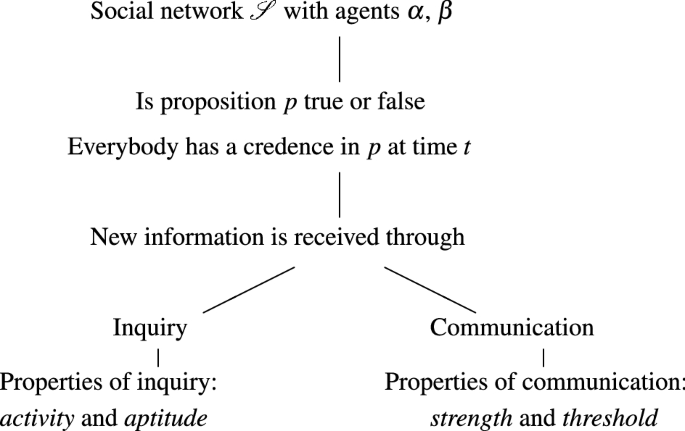Fig. 13

We would like for $$\beta$$ to communicate her actual credence in p to $$\alpha$$, but the precise value might not be available to $$\beta$$, since complete introspection is not possible. Instead we will assume that if $$\beta$$ believes that p, then $$\beta$$ will say p to $$\alpha$$ (and will say $$\lnot p$$ if believes $$\lnot p$$), if $$\beta$$ is not out to mislead $$\alpha$$. The next natural question is then how sure should $$\beta$$ be of p to communicate it to $$\alpha$$ (or how sure of $$\lnot p$$ if $$\beta$$ wants to mislead $$\alpha$$)? Another property of the communication link $$\beta \alpha$$ (besides the strength) is the threshold $$T_{\beta \alpha }$$, which is a value in [0; 1] that depends on $$\beta$$’s credence in p serves as a sort of cut-off point for when $$\beta$$ should tell $$\alpha$$ that p (or that $$\lnot p$$), see Fig. 7 for an illustration. The threshold $$T_{\beta \alpha }$$ fulfills the following conditions according to $$\beta$$ being truthful or misleading (Angere 2010, p. 4):

Truthful::

If $$T_{\beta \alpha }>0.5$$, then $$\beta$$ tells $$\alpha$$ that p only if $$\beta$$’s credence in p is larger than or equal to the threshold; $$C_\beta (p)\ge T_{\beta \alpha }$$, and $$\beta$$ tells $$\alpha$$ that $$\lnot p$$ only if $$\beta$$’s credence in p is less than or equal to 1 minus the threshold (i.e. the credence have to be sufficiently small), $$C_\beta (p)\le 1-T_{\beta \alpha }$$.

Misleading::

If $$T_{\beta \alpha }<0.5$$, then $$\beta$$ tells $$\alpha$$ that p only if $$\beta$$’s credence in p is small, $$C_\beta (p)\le T_{\beta \alpha }$$, and that $$\lnot p$$ only if $$\beta$$’a credence in p is large, $$C_\beta (p)\ge 1-T_{\beta \alpha }$$.

Random::

If $$T_{\beta \alpha =0.5}$$, then $$\beta$$ can tell $$\alpha$$ that p or $$\lnot p$$ independently of what $$\beta$$ believes.

There are two options for altering $$\alpha$$’s credence in p: $$\alpha$$ conducts an inquiry that indicates something (i.e. p or $$\lnot p$$ or neither), or $$\alpha$$ receives communication from someone in the network indicating something (p, $$\lnot p$$ or neither). So inquiry and communication are indications of whether p or $$\lnot p$$ is the case. Our Bayesian framework gives us a way of updating $$\alpha$$’s credence in p when new information is given through inquiry or communication; $$\alpha$$’s credence is updated through Bayesian conditionalization. Figure 13 illustrates the structure of the social network: agents $$\alpha$$ and $$\beta$$ have credences toward the proposition p at all times, and p is assumed to be true in the model. The agents can get new information either by figuring stuff out on their own (inquiry, which can be conducting experiments, reading, thinking, etc.), or by receiving communication by others (communication, which is one person saying either that p or that not-p to another person). The inquiry is characterized by how active the agent is, meaning how often she tries to figure out the truth about p (so this can be though of as a measure of the agent’s curiosity), and by the agent’s aptitude, meaning how good she is at getting the right result when she performs inquiries. Communication is characterized by the strength of the communication link, meaning how often the link is used (this can be thought of as a measure of how chatty the agents are), and by the belief threshold, meaning a bar for how sure an agent has to be in either p or not-p before she can communicate it to someone else.

Before we get to the exact way that $$\alpha$$’s credence is updated, we need to make the notion of an agent receiving information more precise. Collectively, we call an inquiry $$\iota$$ and other agents $$\beta$$, $$\gamma$$, who can talk to $$\alpha$$, sources. To move forward we need a way of knowing how good a source is at indicting the truth. We might call this the reliability of the source $$\sigma$$. The reliability $$R_{\sigma \alpha }$$ of what source $$\sigma$$ tells agent $$\alpha$$ is defined as the probability that $$\sigma$$ indicates (says) p to $$\alpha$$, given that $$\sigma$$ says anything and that p is true (Angere 2010, p. 6):

• Definition:

\begin{aligned} R_{\sigma \alpha }=P(S_{\sigma \alpha } p|S_{\sigma \alpha }\wedge p)= P(S_{\sigma \alpha } \lnot p|S_{\sigma \alpha }\wedge \lnot p) \end{aligned}

So the connection of a source $$\sigma$$ to the truth is given by the probability that $$\sigma$$ gives $$\alpha$$ the right answer.

The last equality means that we assume that the probability of the source telling $$\alpha$$ the correct answer does not depend on the truth of the proposition p. We call this assumption source symmetry. This is an assumption of mathematical convenience, but it is not unreasonable, because intuitively, if you want to tell me the truth about some proposition, it should not matter what that truth is.

An agent does not have access to the true reliability of a source, but can form beliefs about it.

Beliefs about the reliability of a source is expressed as a trust function $$\tau :[0;1]\rightarrow [0;1]$$. So $$\tau _{\sigma \alpha }^t$$ is $$\alpha$$’s trust function for $$\sigma$$ at time t. Define $$\tau _{\sigma \alpha }^t$$ such that the following holds (Angere 2010, p. 6):

\begin{aligned} C_\alpha ^t(a\le R_{\sigma \alpha }\le b)=\int _a^b\tau _{\sigma \alpha }^t(\rho )\, \mathrm {d}\rho \qquad \text {for}\quad a,b\in [0;1] \end{aligned}

This is $$\alpha$$’s credence at time t that the reliability of $$\sigma$$ will be in the interval between a and b given as a density function between a and b. The credence is represented as a density function because the possible values of $$R_{\sigma \alpha }$$ are infinite.

$$\tau _{\sigma \alpha }^t$$ gives the credence-density at $$\rho$$ (the greek letter rho, not the proposition p) and integrating gives $$\alpha$$’s actual credence in propositions about $$\sigma$$’s reliability. Conversely, the expression $$1-\tau _{\sigma \alpha }^t$$ is about $$\alpha$$’s credence in propositions about $$\sigma$$ not being reliable.

### A.2 Updating credence

Before we can get to exactly what $$\alpha$$’s credence-update looks like, we need to introduce a few more assumptions. One is the Principal Principle, which states that if $$\alpha$$ knows that the chance of an event e is $$\rho$$, then $$\alpha$$’s credence in e happening should be exactly $$\rho$$. In the present notation this becomes (Angere 2010, p. 6):

• The Principal Principle (PP):

\begin{aligned} C_\alpha ^t(S_{\sigma \alpha }^t p&|S_{\sigma \alpha }^t \wedge R_{\sigma \alpha }=\rho \wedge p) = \rho \\ C_\alpha ^t(S_{\sigma \alpha }^t \lnot p&|S_{\sigma \alpha }^t \wedge R_{\sigma \alpha }=\rho \wedge \lnot p) = \rho \end{aligned}

More specifically, this formula says that if $$\alpha$$ knows that the reliability of $$\sigma$$ is $$\rho$$, then $$\alpha$$ should assume that the probability that $$\sigma$$ tells the truth is exactly $$\rho$$ (which also amounts to trusting that $$\sigma$$ tells the truth $$\rho \%$$ of the time).

Another assumption to make the modeling easier is Communication Independence, which says that whether or not $$\sigma$$ says anything is independent of the proposition p being true and how reliable $$\sigma$$ is seen to be.

Formally, the assumption says that the propositions p (the proposition under investigation in the network), $$S_{\sigma \alpha }^t$$ (the proposition that the source $$\sigma$$ gives information to $$\alpha$$ at time t), and $$R_{\sigma \alpha }=\rho$$ (the proposition that the reliability of source $$\sigma$$ is $$\rho$$; i.e. $$\sigma$$ gets it right $$\rho$$ percent of the time) are independent, which probabilistically amounts to the following (Angere 2010, p. 7)Footnote 38:

• Communication Independence (CI):

\begin{aligned} C_\alpha ^t(p \wedge S_{\sigma \alpha }^t \wedge R_{\sigma \alpha }=\rho ) = C_\alpha ^t(p) C_\alpha ^t(S_{\sigma \alpha }^t) C_\alpha ^t(R_{\sigma \alpha }=\rho ) \end{aligned}

This is also an assumption of mathematical convenience, since probabilistic independent factors are a lot easier to work with than factors that have underlying dependencies. It is possible to imagine a scenario where $$\sigma$$ is influenced in the choice of whether or not to speak by how reliable $$\alpha$$ thinks $$\sigma$$ is. If I know that you think that I am untrustworthy, then that could persuade me to not speak my mind about p, that is, lower the chance of me communicating with you. What is between the lines of this assumption is that agents only form beliefs about the people that they receive information from (including themselves), not the people they give information to. Practically this means that agents do not change their information depending on who is getting it. In some situations this might be a bit of a stretch, but those could be rare and specific circumstances, such as when there are powerful prejudices involved. If we take this assumption to mean that people can get over their preconceptions and be civil (which does not seem unreasonable), then the assumption is sound.

From PP and CI we can derive the following expression for $$\alpha$$’s credence at time t in $$\sigma$$’s reliability at time t (Angere 2010, p. 7)Footnote 39:

\begin{aligned} C_\alpha ^t(S_{\sigma \alpha }^t p |p) = C_\alpha ^t(S_{\sigma \alpha }^t)\int _0^1\rho \tau _ {\sigma \alpha }^t(\rho )\,\mathrm {d}\rho \end{aligned}

The integral on the right side of the equal-sign is the average, or expected value, of the trust function $$\tau _{\sigma \alpha }^t$$, which we will write as $$\langle \tau _{\sigma \alpha }^t \rangle$$ (Angere 2010, p. 7):

\begin{aligned} \int _0^1\rho \tau _{\sigma \alpha }^t(\rho )\,\mathrm {d}\rho =\langle \tau _{\sigma \alpha }^t \rangle \end{aligned}

So now we have:

This formula says that the degree to which $$\alpha$$ thinks that $$\sigma$$ tells the truth ($$\alpha$$’s credence at time t in the source $$\sigma$$’s testimony that p, given that p is actually true), is given as $$\alpha$$’s credence that the source $$\sigma$$ says something at time t, multiplied with $$\alpha$$’s expected trust in $$\sigma$$’s testimony. We can use ($$*$$) to calculate the credence that $$\alpha$$ should have in p given that $$\sigma$$ tells $$\alpha$$ that p, that is when a source gives $$\alpha$$ new information. This is what we need to determine how $$\alpha$$ should update her credence in p.

\begin{aligned} P_{new}(A) = P(A | E) = \frac{P(E | A)P(A)}{P(E | A)P(A)+P(E | \lnot A)P(\lnot A)} \end{aligned}

In the present situation that we are trying to describe, A is the proposition p, and E is the new evidence, which is the information that $$\sigma$$ says that p: $$S_{\sigma \alpha }^t p$$. $$P_{new}(A)$$ is the updated credence that $$\alpha$$ should have in p, which we will write as $$C^{t+1}_{\alpha }(p)$$. We can now calculate $$\alpha$$’s updated credence in p (Angere 2010, p. 7):

\begin{aligned} C^{t+1}_{\alpha }(p) = C_\alpha ^t(p |S_{\sigma \alpha }^t p)&= \frac{C_\alpha ^t(S_{\sigma \alpha }^t p |p) C_\alpha ^t(p)}{C_\alpha ^t(S_{\sigma \alpha }^t p |p)C_\alpha ^t(p) + C_\alpha ^t(S_{\sigma \alpha }^t p |\lnot p)C_\alpha ^t(\lnot p)}\\&= \frac{C_\alpha ^t(S_{\sigma \alpha }^t) \langle \tau _{\sigma \alpha }^t \rangle C_\alpha ^t(p)}{C_\alpha ^t(S_{\sigma \alpha }^t) \langle \tau _{\sigma \alpha }^t \rangle C_\alpha ^t(p) + C_ \alpha ^t(S_{\sigma \alpha }^t) \langle 1-\tau _{\sigma \alpha }^t \rangle C_\alpha ^t(\lnot p)}\\&= \frac{\langle \tau _{\sigma \alpha }^t \rangle C_\alpha ^t(p)}{\langle \tau _{\sigma \alpha }^t \rangle C_\alpha ^t(p) + \langle 1-\tau _{\sigma \alpha }^t \rangle C_\alpha ^t(\lnot p)} \end{aligned}

This formula says that $$\alpha$$’s credence in p given that the source $$\sigma$$ says that p can be calculated as $$\alpha$$’s initial credence in p times $$\alpha$$’s expected trust in $$\sigma$$ (which basically means “how likely does $$\alpha$$ think it is that $$\sigma$$ is right”), divided by the probability that $$\sigma$$ is right plus the probability that $$\sigma$$ is wrong. We can derive a similar formula for the case where the source $$\sigma$$ says that not-p ($$S_{\sigma \alpha }^t \lnot p$$) (Angere 2010, p. 7):

\begin{aligned} C_\alpha ^t(p |S_{\sigma \alpha }^t \lnot p) = \frac{\langle 1- \tau _{\sigma \alpha }^t \rangle C_\alpha ^t(p)}{\langle 1- \tau _{\sigma \alpha }^t \rangle C_\alpha ^t(p) + \langle 1-\tau _{\sigma \alpha }^t \rangle C_\alpha ^t(\lnot p)} \end{aligned}

When $$\sigma$$ is the only source giving information to $$\alpha$$ at time t, this formula completely determines how $$\alpha$$ should update her credence in p.

Note that when an agent reaches full belief (credence 0 or 1), they cannot change their mind again. To see this, consider the formula for updating agent $$\alpha$$’s belief in light of new information from source $$\sigma$$ (cf. Sect. 3.2):

\begin{aligned} C^{t+1}_{\alpha }(p) = \frac{\langle \tau _{\sigma \alpha }^t \rangle C_\alpha ^t(p)}{\langle \tau _ {\sigma \alpha }^t \rangle C_\alpha ^t(p) + \langle 1-\tau _{\sigma \alpha }^t \rangle C_\alpha ^t(\lnot p)} \end{aligned}

In the case where $$\alpha$$ has full belief in p, that is, where $$C_\alpha ^t(p)=1$$ and $$C_\alpha ^t(\lnot p)=0$$, this formula gives us:

\begin{aligned} C^{t+1}_{\alpha }(p) = \frac{\langle \tau _{\sigma \alpha }^t \rangle \times 1}{\langle \tau _{\sigma \alpha }^t \rangle \times 1 + \langle 1-\tau _{\sigma \alpha }^t \rangle \times 0} =\frac{\langle \tau _ {\sigma \alpha }^t \rangle }{\langle \tau _{\sigma \alpha }^t \rangle }=1 \end{aligned}

And when $$\alpha$$ has full belief in $$\lnot p$$, that is, when $$C_\alpha ^t(p)=0$$, the numerator of the fraction becomes 0, so the result of the updated belief remains 0. In either case, $$\alpha$$’s belief cannot be changed when it has reached the extremes.

In the updating formula, there are two key parts; the trust $$\langle \tau _{\sigma \alpha } \rangle$$ and the message expectation $$C_{\alpha }^t(p)$$. Naturally, the value of these components influence whether $$\alpha$$’s credence in p is strengthened or weakened. We can describe the components in the following way (Angere 2010, p. 7):

• The source $$\sigma$$ is trusted if $$\langle \tau _{\sigma \alpha }^t \rangle > 0.5$$.

• The source $$\sigma$$ is distrusted if $$\langle \tau _{\sigma \alpha }^t \rangle < 0.5$$.

• The source $$\sigma$$ is neither trusted nor distrusted if $$\langle \tau _{\sigma \alpha }^t \rangle = 0.5$$.

• The message m is expected if $$C_{\alpha }^t(p) > 0.5$$ and $$m=p$$ or if $$C_{\alpha }^t(p) < 0.5$$ and $$m= \lnot p$$ (that is, if the message confirms what $$\alpha$$ already believes).

• The message m is unexpected if $$C_{\alpha }^t(p) > 0.5$$ and $$m= \lnot p$$ or if $$C_{\alpha }^t(p) < 0.5$$ and $$m= p$$ (that is, if the message goes against what $$\alpha$$ already believes).

• The message m is neither expected nor unexpected if $$C_{\alpha }^t(p) = 0.5$$.

### A.3 Several sources

The above calculations are for a situation where $$\alpha$$ only is getting information from a single source $$\sigma$$. But we would like to be able to generalize to a situation where $$\alpha$$ can receive multiple messages at the same time, so that we can model the dynamic of a group of people.

Let $$\Sigma _\alpha ^t$$ be the set of sources from which $$\alpha$$ receives a message about p at time t. Let $$m_{\sigma \alpha }^t$$ be the message from $$\sigma$$ to $$\alpha$$, which can be either p or $$\lnot p$$. We want a way to express $$\alpha$$’s credence in p given that $$\alpha$$ receives several messages from as many sources.

From conditionalization we have that for n different sources $$\sigma _1$$ to $$\sigma _n$$ the following formula holds (Angere 2010, p. 8):

\begin{aligned} C_\alpha ^{t+1}(p)&= C_\alpha ^t(p|S_{\sigma _1 \alpha }^t m_{\sigma _1 \alpha }^t \wedge S_{\sigma _2 \alpha }^t m_{\sigma _2 \alpha }^t \wedge \cdots \wedge S_{\sigma _n \alpha }^t m_{\sigma _n \alpha }^t)\\&=C_\alpha ^t(p |\bigwedge S_{\sigma \alpha }^t m_{\sigma \alpha }^t) \end{aligned}

This says that when $$\alpha$$ gets information from many sources at one time, then $$\alpha$$’s updated credence in p is calculated as the original credence in p given the conjunction of all the messages from the different sources. So the updated credence in p is the initial credence in p conditional on the information from source 1, and the information from source 2, and so on. The conjunction runs over all sources $$\sigma$$ in $$\Sigma _\alpha ^t$$. In order to make the complexity of this expression manageable, we will assume that agents treat their sources as independent, given the truth or falsity of p.

Formally this means that we assume source independenceFootnote 40 (SI) (Angere 2010, p. 8):

\begin{aligned} C_\alpha ^t\left( \bigwedge S_{\sigma \alpha }^t m_{\sigma \alpha }^t |p \right)&= C_\alpha ^t(S_{\sigma _1\alpha }^t m_{\sigma _1\alpha }^t |p)\times \cdots \times C_\alpha ^t(S_{\sigma _n\alpha }^t m_{\sigma _n\alpha }^t |p)\\&=\prod C_\alpha ^t(S_{\sigma \alpha }^t m_{\sigma \alpha }^t |p) \end{aligned}

Although it might not always be the case that sources are independent it is a reasonable assumption to make for simplicity. After all, people do not generally try to detect the underlying dependencies of their sources.

Now we can derive how agents should update their credence given new information from several sources. From source independence, the properties of the individual communication links and the agents we can get the following from Bayes’ theorem (Angere 2010, p. 8)Footnote 41:

\begin{aligned}&C_\alpha ^t(p |\bigwedge S_{\sigma \alpha }^t m_{\sigma \alpha }^t) \\&\quad =\frac{C_\alpha (p) \prod C_\alpha ^t(S_{\sigma \alpha }^t m_{\sigma \alpha }^t |p)}{C_\alpha (p) \prod C_\alpha ^t(S_{\sigma \alpha }^t m_{\sigma \alpha }^t |p) + C_\alpha (\lnot p) \prod C_\alpha ^t(S_{\sigma \alpha }^t m_{\sigma \alpha }^t |\lnot p)} \end{aligned}

The values of $$C_\alpha ^t(S_{\sigma \alpha }^t m_{\sigma \alpha }^t|p)$$ and $$C_\alpha ^t(S_{\sigma \alpha }^t m_{\sigma \alpha }^t|\lnot p)$$ are determined by the equation $$(*)$$ above, which means we have all the necessary information to determine exactly what degree of belief $$\alpha$$ should have in p given the information she receives at time t.

### A.4 Updating trust function

Even though people do not expect dependencies between their sources, they do keep track of how reliable the sources are. A source that keeps reporting unexpected messages is seen as unlikely to be correct, meaning that people are less likely to trust that source with regards to p (in reality, probably also with regards to other propositions, but that is beyond the scope of this model). So we want a way to adjust $$\alpha$$’s trust function in light of the messages that the source gives.

In the model that is done in the following way: $$\alpha$$’s trust function for the source $$\sigma$$ at time t is $$\tau _{\sigma \alpha }^t$$. If $$\alpha$$ receives the message that p from $$\sigma$$ then $$\alpha$$’s new trust function $$\tau _{\sigma \alpha }^{t+1}$$ is calculated by the following formula (Angere 2010, p. 9):

\begin{aligned} \tau _{\sigma \alpha }^{t+1}(\rho ) = \tau _{\sigma \alpha }^t(\rho ) \frac{\rho C_\alpha ^t(p) + (1-\rho ) C_\alpha ^t(\lnot p)}{\langle \tau _{\sigma \alpha }^t \rangle C_\alpha ^t(p) + \langle 1-\tau _{\sigma \alpha }^t \rangle C_\alpha ^t(\lnot p)} \end{aligned}

Or, if $$\sigma$$ gives the message that $$\lnot p$$, then the updated trust function is given by (Angere 2010, p. 9)Footnote 42:

\begin{aligned} \tau _{\sigma \alpha }^{t+1}(\rho ) = \tau _{\sigma \alpha }^t(\rho ) \frac{\rho C_\alpha ^t(\lnot p) + (1-\rho ) C_\alpha ^t(p)}{\langle \tau _{\sigma \alpha }^t \rangle C_\alpha ^t(\lnot p) + \langle 1-\tau _{\sigma \alpha }^t \rangle C_\alpha ^t(p)} \end{aligned}

The above model is employed in a computer program called Laputa which facilitates studying the kinds of Bayesian social networks that we are talking about.Footnote 43

### B.1 The parameters

In Laputa, there are several ways of simulating the belief evaluation of a group of Bayesian agents. One of the more useful ways is the batch simulation, which allows you to define the general traits of the population and collect statistics over many trials. As Laputa is based on a probabilistic model, this is the only type of simulation that can provide enough information to say something general about a population (that is, single simulations can only give information about that specific scenario). The traits of the population are determined by several parameters that are given as probability distributions. That is, when you set the parameters, you set the probabilities that the population will behave in a certain way. The parameters are the following:

• Link density Distribution of the probability that a given agent will have a number of communication channels to other agents in the network. It describes how many of the agents in the network have the possibility of communicating.

• Starting belief Distribution of the initial beliefs of the agents in the network, before the agents have received any information about p.

• Inquiry accuracy Distribution of the probability that agents in the network get the right result from inquiry. It describes the aptitude of the group of finding out things about p on their own.

• Inquiry chance Distribution of the probability that agents in the group will engage in inquiry at any given time step. It describes how active, or curious, the group is.

• Inquiry trust Distribution of the probability that a given agent’s inquiry trust function will have a certain shape. Since each agent in the network has a probability distribution of inquiry trust (describing the chance that the agent will trust her own inquiry at a given time step), this is actually a probability distribution of the probability distributions for every agent in the network. It describes how good the agents think they are at getting the right result from inquiry.

• Communication chance Distribution of the probability that agents in the network will engage in communication at any given time step, that is, the chance that they will say something to someone else in the network. It describes how chatty agents are in the network.

• Communication trust This distribution is similar to the inquiry trust distribution, in that it is a distribution of distributions that say something about the chance that a given agent will trust what someone else tells them at a given time step. It describes how goos the agents think others are at telling the truth.

• Belief threshold: Distribution of the credences the agents need to have in p or $$\lnot p$$ before they can communicate it to others.

There are also a few parameters not given as probability distributions, which are:

• Number of agents This can be either a fixed number, or the program can be set to generate groups of varying sizes.

• New evidence requirement This specifies whether an agent needs new information before she can communicate again. It can be seen as describing a sort of confirmation necessity, in that agents need to get extra information before they can repeat their opinion to someone. The new evidence requirement is either that the agent conducts an inquiry, or receives information from someone else, or either of the above.

• Number of steps This can be thought of as a time scale, but every step represents one round of agents conducting an inquiry and giving a piece of information through communication channels. Every piece of information that an agent receives changes her credence in p and her trust function (see “Appendix A” for details). So every time step describes a short group deliberation and a private belief update for every agent in the network (private in the sense that agents’ credences are not available to other agents in the network).

• Number of trials This determines how many times the simulation is run, which means that one can get statistically significant data about the group that one is investigating.

### B.2 The population

In the experiment, we are looking at groups having 50 members, where everybody can communicate with somebody else, meaning that for a given agent there can be between 1 and 49 links to other agents (determined by the link density parameter). This means that there is a possibility of communication for everybody, but whether communication actually occurs is determined by the parameters communication chance and belief threshold. The communication chance parameter is set to be random (modeled by a uniform probability distribution), so a given agent’s chance of communication might as well be 0.2 as 0.99 (or any other number between 0 and 1). The belief threshold is set to be uniformly distributed, except at 0.5 and surrounding values (for a degree of belief in p between 0.469 and 0.531 the agent cannot communicate), essentially meaning that an agent just has to be more sure than random to communicate. As it is assumed in the model that p is true, it might seem counter-intuitive that the belief threshold shouldn’t be higher. But what the belief threshold parameter actually tells us is the lowest credence an agent can have in p in order to claim that p, and the highest threshold in order to claim that $$\lnot p$$. Since the point of this experiment is to investigate effects on polarization, it is important that agents that start out believing $$\lnot p$$ can speak their mind with the same probability as someone who start out believing p.

The inquiry accuracy is set to be a normal distribution with mean 0.6 and standard deviation 0.15. This means that most of the agents get the right result from inquiry 60% of the time, and that the other agents’ accuracies are reasonably close to this value. We can interpret this as being a fairly competent population, that is, on average they get the right answer more than they get the wrong answer from inquiry. The inquiry chance is set to be uniformly distributed, so there is as much chance for an agent to be very active as very lazy. There is no new evidence requirement, meaning that agent’s do not necessarily have to get new information in order to communicate their beliefs again. The simulations are set to run over 30 steps, which can be thought of as a pretty long time scale; it correspond to 30 different instances of group deliberation and belief revision. The data was collected and averaged over 20,000 iterations.

### B.3 The groups

We are looking at five different distributions of starting beliefs, representing five different types of groups. These are groups that are either undecided about p, generally agree that p is true or that disagree whether p or $$\lnot p$$ is true. This last option is possible by Laputa’s freeform distribution option, where one can draw the distributions of starting beliefs by hand (see Fig. 2 for diagrams from Laputa of the different starting beliefs). The distributions for the five groups are as follows:

• The undecided group Normal distribution with mean 0.5 and standard deviation 0.15. This is a group that is generally undecided as to the veracity of p.

• The polarized group Freeform distribution with tops at 0.406 and 0.594. This group is polarized, where most believe either p or $$\lnot p$$, but not with very strong conviction. A few are undecided and none start out with absolute conviction (which would be credence 1 or 0).

• The very polarized group Freeform distribution with tops at 0.297 and 0.703. This is also a polarized group, but where people have stronger convictions as to whether or not p is true. Again, a few are undecided and none start out with absolute conviction.

• The suspecting group Normal distribution with mean 0.65 and standard deviation 0.15. This is a group that generally believe more in p than $$\lnot p$$, but not with strong conviction.

• The very suspecting group Normal distribution with mean 0.75 and standard deviation 0.15. This is a group that generally have even stronger beliefs in the truth of p than group $$\delta$$.

For each of these groups we looked at three different developments of the inquiry trust and communication trust distributions. The point of these three different developments is to see if a change in the way you trust yourself, compared to how much you trust others, has an effect on the end polarization of these different groups. The trust distribution developments are characterized in the following way (all distributions are normal distributions and the standard deviation is 0.2 for all simulations):

Inquiry trust equals communication trust Inquiry trust and communication trust are equal, and the mean values of both the inquiry and the communication trust is randomly picked from a small interval. We document the polarization behavior of each of the five group for 19 different intervals, that cover a wide variety of possible attitudes toward evidence. In the first interval means of trust distribution are between 0.1 and 0.2, which means that agents can trust themselves and others between 10 and 20% of the time. The intervals then move slowly closer to 1 (each interval has the same size), ending in an interval between 0.8 and 0.9. This illustrates a development of increasing trust, where you at all times trust others roughly the same as you trust yourself.

Inquiry trust is stable and communication trust varies Inquiry trust is kept fixed and communication trust takes many different values. For inquiry trust the mean is randomly picked between 0.5 and 0.6, that is, whether you trust your own inquiry is just slightly better than letting a coin decide if you should trust yourself. The mean value of the communication trust is picked from a small interval, in the same fashion as above. In the first interval the mean value of the communication trust distribution is between 0.1 and 0.2, and this interval is moved closer to 1 over 19 different intervals, which represent a wide variety of attitudes toward testimony from others. This illustrates a situation where you distrust the testimony of others in a wide variety of ways, and keep a moderate faith in yourself. These trust distributions apply to the first stage in the simulation. The trust function is updated along with the credence in p for each step in the simulation.

Inquiry trust varies and communication trust is stable Communication trust is kept fixed and inquiry trust takes many different values. This development behaves analogously to the development where the inquiry trust is stable and the communication trust varies. The mean of the communication trust is picked randomly between 0.5 and 0.6, that is, agents start out with a moderate trust in others. The mean of the inquiry trust starts out being picked randomly from the interval between 0.1 and 0.2 and moves closer to 1 in the same way as above. This illustrates a situation where agents have many different attitudes toward their own ability, and they start out with moderate faith in the testimony of others.

These three developments gives rise to three different diagrams for each of the five groups (see Fig. 9). Each diagram shows how polarized each of the five groups are after 30 time steps, for each of the intervals of the mean values of the trust distributions.

## Rights and permissions

Reprints and Permissions